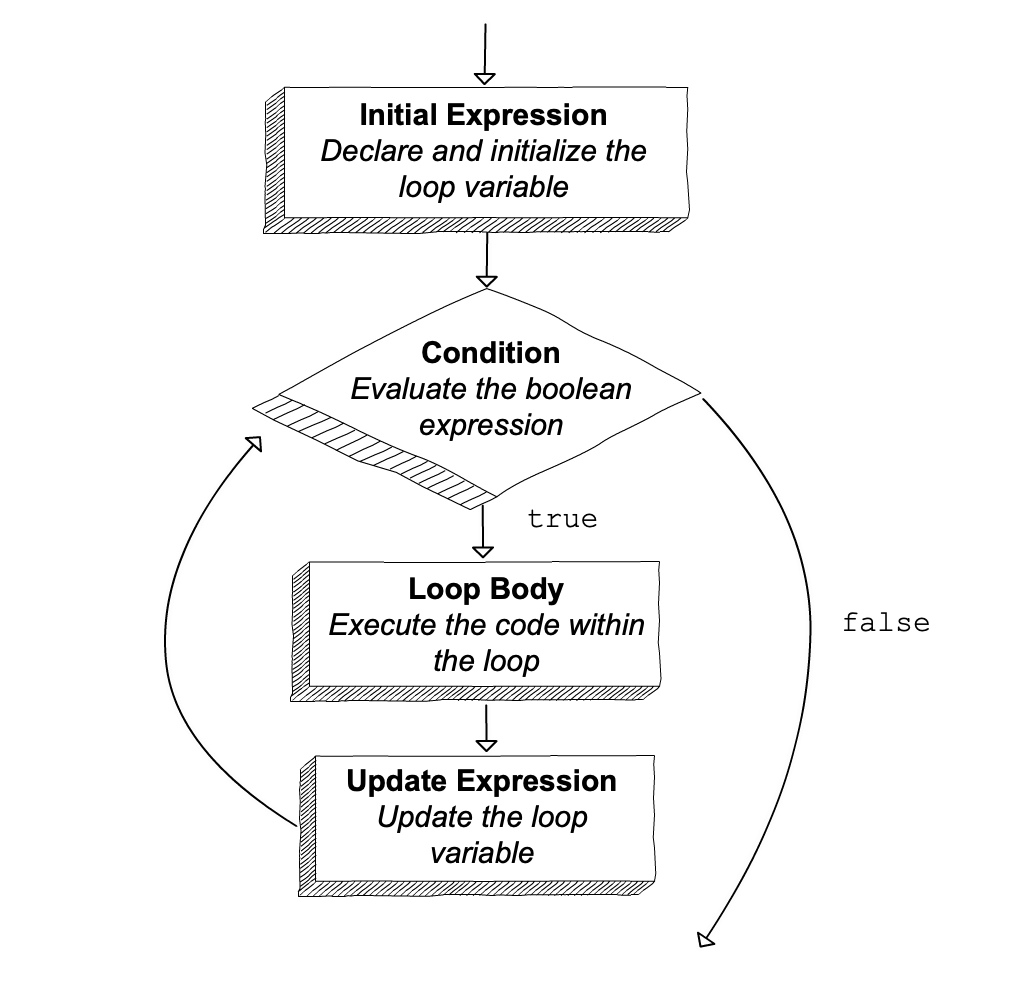# for Loops

The `for` loop is the first JavaScript tool for iteration that we will explore. A for loop is typically used for definite iteration. Definite iteration is the process of repeating a specific task with a specific data set. When a `for` loop begins it can usually be determined exactly how many times it will execute: once for each item in the data set.

## `for` Loop Syntax

We have already seen the basic syntax of a `for` loop.

 ``````1 2 3 `````` ``````for (let i = 0; i < 51; i++) { console.log(i); } ``````

This program prints the integers 0 through 50, one number per line. In the language of definite iteration, we say that the loop has a data set of 0-50, and its action is to print a value to the console.

Let’s break down this syntax piece by piece, so we can begin to understand how `for` loops are structured.

A `for` loop always contains the following components:

``````for (initial expression; loop condition; update expression) {
loop body
}
``````

Notice that in the first line, within parentheses, the components initial expression, loop condition, and update expression are separated by semicolons. Let’s look at these components in detail.

1. The statement `let i = 0` is executed exactly once, at the beginning of loop execution. The variable `i` is the loop variable.

2. The boolean expression `i < 51` is the loop condition. This condition is evaluated before each loop iteration, or repetition.

• If the condition is `true` then the loop executes again.
• If the condition is `false` then the loop ceases execution, and the program moves on to the code below the loop.
3. The statement `i++` is the update expression. This expression is executed at the end of each loop iteration.

4. The block of code surrounded with brackets (`{ }`) is the loop body. The body is executed once for each iteration of the loop.

## Flow of Execution of the `for` Loop

In just a few lines of code, a `for` loop contains a lot of detailed logic, so let’s spend some time breaking down the flow of execution for the particular loop that we’ve been looking at.

 ``````1 2 3 `````` ``````for (let i = 0; i < 51; i++) { console.log(i); } ``````

Here is a step-by-step description of how this loop executes:

1. When the program reaches the `for` loop, the initial expression `let i = 0` is executed, declaring the variable `i` and initializing it to the value `0`.
2. The loop condition `i < 51` is evaluated, returning `true` because 0 is less than 51.
3. Since the condition is `true`, the loop body executes, printing 0.
4. After the execution of the loop body, the update expression `i++` is executed, setting `i` to 1. This completes the first iteration of the loop.
5. Steps 2 through 4 are repeated, using the new value of `i`. This continues until the loop condition evaluates to `false` in step 2, ending the loop. In this example, this occurs when `i < 51` is `false` for the first time. Since our update expression adds 1 after each iteration, this occurs when `i` is 51 (so `51 < 51` is `false`). At that point, the loop body will have executed exactly 51 times, with `i` having the values 0…50.

In general, we can visualize the flow of execution of a `for` loop as a flowchart.